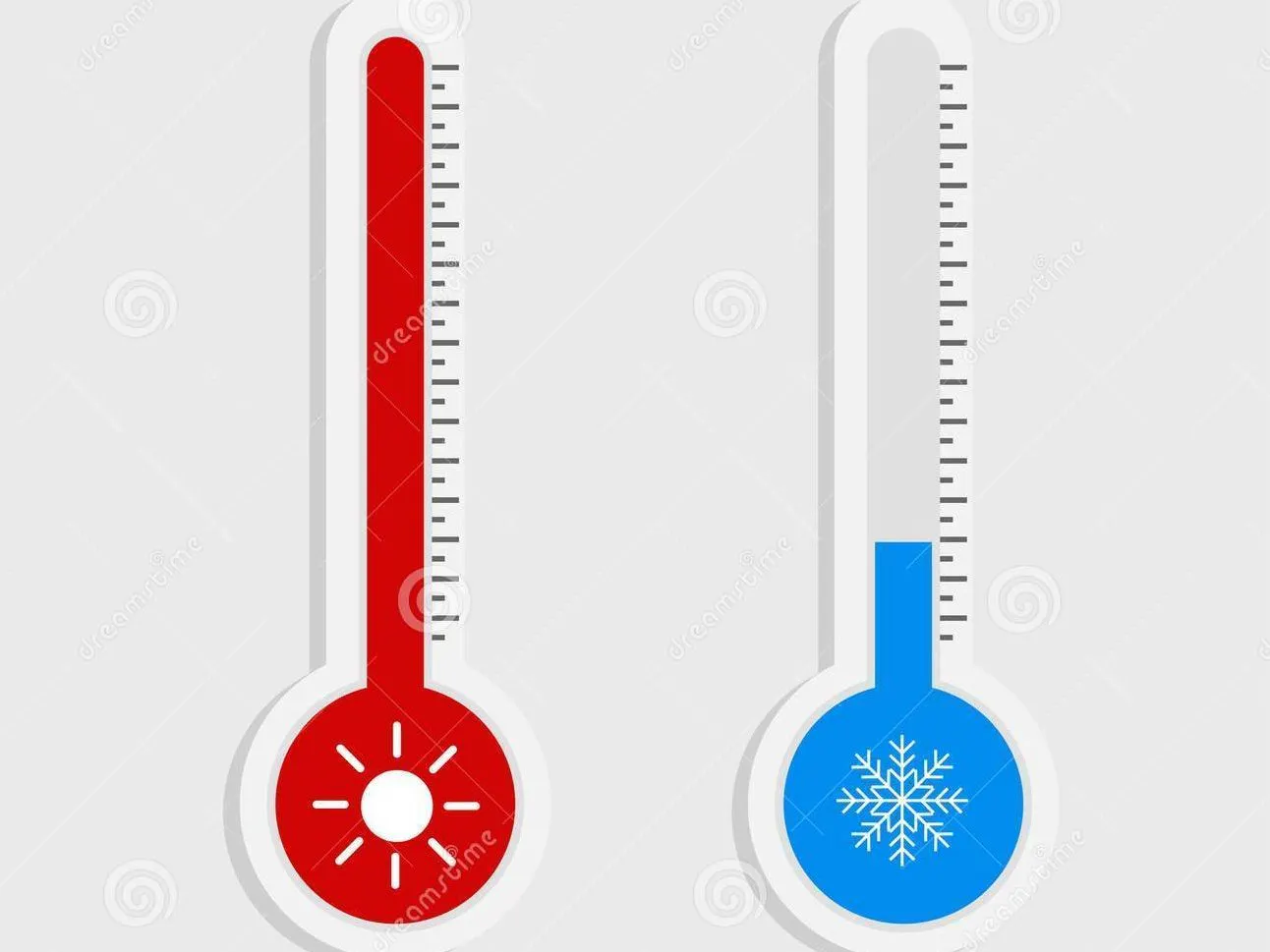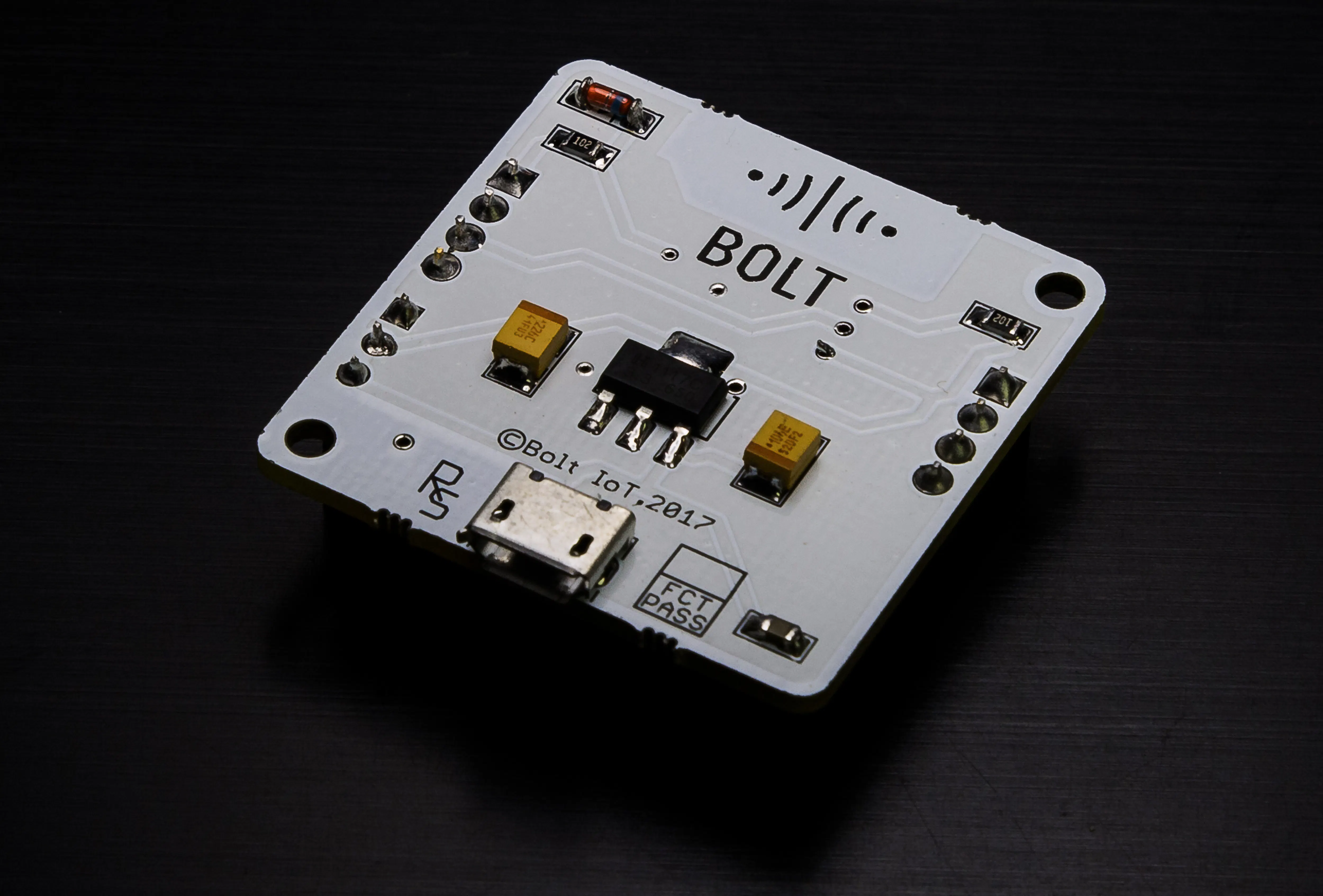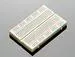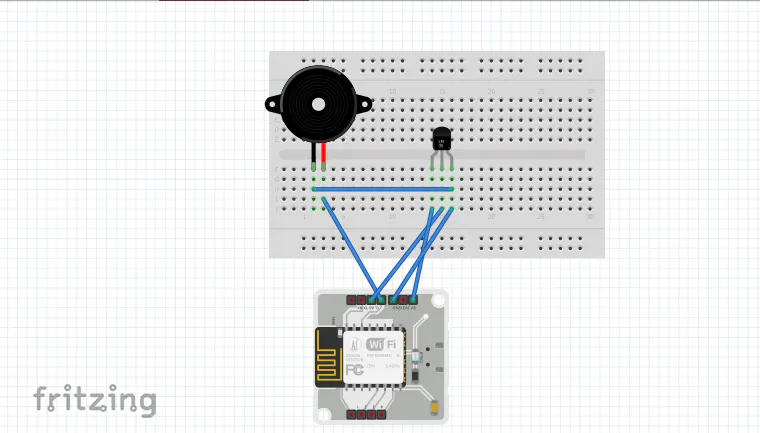Created July 8, 2020

# Temperature Monitoring and Alert System!!

Monitors if the temperature of the environment is within the authorized range and sends an alert in case of any anomaly detection.

IntermediateFull instructions provided4## Things used in this project

### Hardware componentsBolt IoT Bolt WiFi Module
×1Temperature Sensor
×1Jumper wires (generic)
×1×1Buzzer
×1
 Power bank
×1

### Software apps and online servicesTwilio SMS Messaging APIBolt IoT Bolt Cloud
 Ubuntu 16.04
 Mailgun

## Schematics

### Schematic## Code

### Anomaly detection using Z-Score Analysis

Python
Detect an anomaly in the temperature and alert via a SMS and buzzer
```import conf, json, time, math, statistics
from boltiot import Sms, Bolt

def compute_bounds(history_data, frame_size, factor): #determine the bounds dynamically
if len(history_data)<frame_size:
return None
if len(history_data)>frame_size:
del history_data[0:len(history_data)-frame_size]
Mn= statistics.mean(history_data)
Variance=0
for data in history_data:
Variance += math.pow((data-Mn),2)
Zn = factor * math.sqrt(Variance / frame_size)
High_bound= history_data[frame_size-1]+Zn
Low_bound= history_data[frame_size-1]-Zn
return [High_bound, Low_bound]

mybolt= Bolt(conf.API_KEY, conf.DEVICE_ID) # to communicate with the bolt cloud and fetch values
sms = Sms(conf.SSID, conf.AUTH_TOKEN, conf.TO_NUMBER, conf.FROM_NUMBER) # instance to send an sms when anomaly occurs
history_data=[]

while True:

data = json.loads(response) # response in JSON format, hence use python json library to load the data
if data['success']!=1:
print("There was an error while retriving the data.")
print("This is the error:" +data['value'])
time.sleep(10)
continue
print("THis is the value " +data['value'])

sensor_value=0
try:
sensor_value = int(data['value'])
except e:
print("There was an error while parsing the response:", e)
bound= compute_bounds(history_data, conf.FRAME_SIZE, conf.MUL_FACTOR)
if not bound:
required_data_count= conf.FRAME_SIZE-len(history_data)
print("Not enough data to compare the Z score. Need", required_data_count,"more data points")
history_data.append(int(data['value'])) # append the data to the previously collected data
time.sleep(10)
continue

try:
if sensor_value>bound or sensor_value<bound: #check for anomaly
print("The temperature level changed suddenly. ending an sms")
response = sms.send_sms("Someone has opened the fridge")
print("This is the response",response)
res=mybolt.analogWrite(0,250) #set the buzzer high for 50 secs
print(res)
time.sleep(50)
res=mybolt.analogWrite(0,0)
print(res)
history_data.append(sensor_value);
except Exception as e:
print("Error",e)
time.sleep(10)
```

### Polynomial Regression

JavaScript
To predict the future temperatures
```setChartLibrary('google-chart');
setChartTitle('Polynomial Regression');
setChartType('predictionGraph');
setAxisName('time_stamp','temp');
mul(0.0977); // temperature(in celsius)= (100*sensor value) / 1024
plotChart('time_stamp','temp');
```

### Temperature alert system using defined threshold

Python
Send an alert via mail when the temperature exceeds the threshold we set
```import email_conf, json, time
from boltiot import Bolt,Email
maximum_limit=103
minimum_limit=0
mybolt=Bolt(email_conf.API_KEY, email_conf.DEVICE_ID)
mailer= Email(email_conf.MAILGUN_API_KEY, email_conf.SANDBOX_URL, email_conf.SENDER_EMAIL, email_conf.RECIPIENT_EMAIL)
while True:
print("sensor value is" + str(data['value']))
try:
sensor_value= int(data['value'])
if sensor_value>maximum_limit or sensor_value<minimum_limit:
print("Making request to mailgun to send an email")
response= mailer.send_email("Alert","The current temperture sensor value is" + str(sensor_value))
print("Response received from Mailgun is:" + str(response_text['message']))
except Exception as e:
print("Error occured: below are the details")
print(e)
time.sleep(10)
```

## Credits

### Tejaswini Panati

0 projects • 0 followers